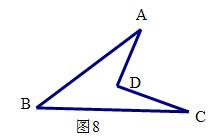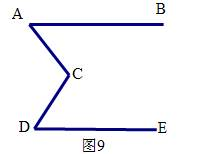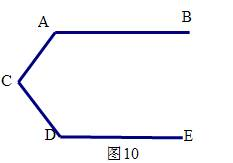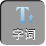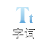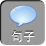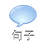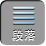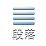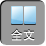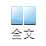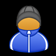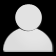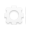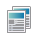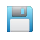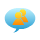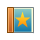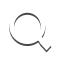-AA+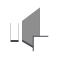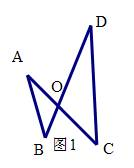1、如图 2，求∠ A+∠ B+∠ C+∠ D+∠ E的度数。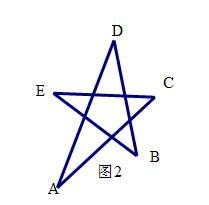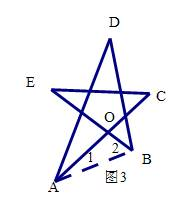E+∠ C=∠ 1+∠ 2

∴∠ A+∠ B+∠ C+∠ D+∠ E=∠ 1+∠ 2+∠ DAO+∠ DBO+∠ D

1+∠ 2+∠ DAO+∠ DBO+∠ D= 1800

∴∠ A+∠ B+∠ C+∠ D+∠ E= 1800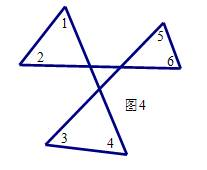1、如图 5，你能得出∠ B、∠ A、∠ C、∠ ADC之间的关系吗？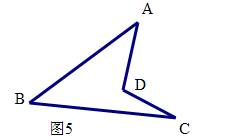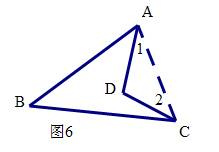B+∠ BAC+∠ ACB= 1800

∴∠ B+∠ BAD+∠ 1+∠ DCB+∠ 2= 1800

1+∠ ADC+∠ 2= 1800

∴∠ 1+∠ ADC+∠ 2=∠ B+∠ BAD+∠ 1+∠ DCB+∠ 2

∴∠ ADC=∠ B+∠ BAD+∠ DCB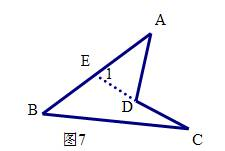∵∠ ADC是⊿ AED的一个外角

∴∠ ADC=∠ 1+∠ A

∵∠ 1是⊿ BEC的一个外角

∴∠ 1=∠ B+∠ C

∴∠ ADC=∠ B+∠ A+∠ C